Ask your WordPress questions! Pay money and get answers fast!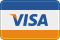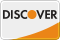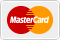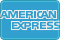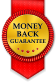# Filtering query by last 7 days WordPress

• SOLVED

This is code from a popular posts plugin on my wordpress site

```\$query = "SELECT post_id FROM \$wpdb->postmeta WHERE meta_key = 'views_count' AND post_id IN (".\$allpostsids.") ORDER BY meta_value*1 ".\$orden." LIMIT ".\$limit; ```

I need to alter this so that it only gets posts from the last 7 days

2013-12-14

Hello,

Can you show the query for fetching `\$allpostsids`. Need to add filter in that query.

Provide me the details of plugin that you are using.

Here is the code above it, hopefully this will help

``` // Checking the order / Comprobando el orden \$orden = strtoupper(\$order); if(\$orden != "DESC" && \$orden != "ASC") \$orden = "DESC"; // Checking the num >= 0 / Comprobando que el nÃºmero sea >= 0 \$limit = \$num; if(\$limit < 0) \$limit = 0; // Checking \$type is a valid post type / Comprobando que el tipo sea un tipo vÃ¡lido \$posttype = \$type; \$post_types = get_post_types(); if(!in_array(\$posttype,\$post_types)) \$posttype = "post"; // Gets all IDs from \$type / Recuperamos todas las IDs de ese tipo. \$args = array( "post_type"=>\$posttype, "numberposts"=>-1 ); \$all_posts = get_posts(\$args); \$postids = array(); foreach(\$all_posts as \$onepost){ \$postids[] = \$onepost->ID; } \$allpostsids = implode(",",\$postids);```

Change `\$all_posts = get_posts(\$args);` with following code

``` // Create a new filtering function that will add our where clause to the query function filter_where( \$where = '' ) { // posts in the last 7 days \$where .= " AND post_date > '" . date('Y-m-d', strtotime('-7 days')) . "'"; return \$where; } add_filter( 'posts_where', 'filter_where' ); \$all_posts = get_posts(\$args); remove_filter( 'posts_where', 'filter_where' ); ```

Unfortunately that did not do the trick. It made no difference

`\$all_posts = get_posts(\$args);`
`\$all_posts = query_posts(\$args);`